Jaccard similarity and Jaccard distance in Python

0
56In this tutorial we will explore how to calculate the Jaccard similarity (index) and Jaccard distance in Python.

Introduction

Jaccard similarity (Jaccard index) and Jaccard index are widely used as a statistic for similarity and dissimilarity measurement. Their applications ranges from simple set similarities, all the way up to complex text files similarities.

To continue following this tutorial we will need the following Python libraries: scipy, sklearn and numpy.

If you don’t have it installed, please open “Command Prompt” (on Windows) and install it using the following code:

pip install scipy
pip install sklearn
pip install numpy

What is Jaccard similarity

The Jaccard similarity (also known as Jaccard similarity coefficient, or Jaccard index) is a statistic used to measure similarities between two sets.

Its use is further extended to measure similarities between two objects, for example two text files. In Python programming, Jaccard similarity is mainly used to measure similarities between two sets or between two asymmetric binary vectors.

Mathematically, the calculation of Jaccard similarity is simply taking the ratio of set intersection over set union.

Consider two sets A and B :Then their Jaccard similarity (or Jaccard index) is given by: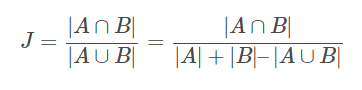Let’s break down this formula into two components:

1. Nominator

The nominator is effectively the set intersection between A and B , shown by the yellow area in the infographic below:2. Denominator

The denominator is effectively the set union of A and B , shown by the yellow area in the infographic below:Using the formula of Jaccard similarity, we can see that the similarity statistic is simply the ratio of the above two visualizations, where:

• If both sets are identical, for example
$(A = {1, 2, 3})$

$(B = {1, 2, 3})$

• If sets A and B don’t have common elements, for example, say
$(A = {1, 2, 3})$

$(B = {4, 5, 6})$

• If sets sets A and B have some common elements, for example,
$(A={1,2,3})$

$(B = {3, 4, 5})$

$(0 leq J(A, B) leq 1)$

Calculate Jaccard similarity

Consider two sets:

• A = {1, 2, 3, 5, 7}
• B = {1, 2, 4, 8, 9}

Or visually:Step 1:

As the first step, we will need to find the set intersection between A and B :In this case:

$A cap B = {1, 2}$

Step 2:

The second step is to find the set union of A and B :In this case:

$A cup B = {1, 2, 3, 5, 7, 4, 8, 9}$

Step 3:

And the final step is to take the ratio of sizes of intersection and union:

$J = frac{|A cap B|}{|A cup B|} = frac{2}{8} = 0.25$

What is Jaccard distance

Unlike the Jaccard similarity (Jaccard index), the Jaccard distance is a measure of dissimilarity between two sets.

Mathematically, the calculation of Jaccard distance is the ratio of difference between set union and set intersection over set union.

Consider two sets A and B :Then their Jaccard distance is given by: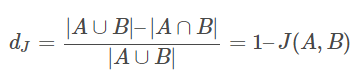Let’s break down this formula into two components:

1. Nominator

The nominator can be also written as: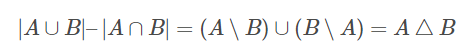which is effectively the set symmetric difference between A and B , shown by the yellow area in the infographic below:2. Denominator

The denominator is effectively the set union of A and B , shown by the yellow area in the infographic below:Using the formula of Jaccard distance, we can see that the dissimilarity statistic is simply the ratio of the above two visualizations, where:

• If both sets are identical, for example
$(A = {1, 2, 3})$

$(B = {1, 2, 3})$

• If sets A and B don’t have common elements, for example, say
$(A = {1, 2, 3})$

$(B = {4, 5, 6})$

• If sets sets A and B have some common elements, for example,
$(A={1,2,3})$

$(B = {3, 4, 5})$

$(0 leq d_J(A, B) leq 1)$

Calculate Jaccard distance

Consider two sets:

• A = {1, 2, 3, 5, 7}
• B = {1, 2, 4, 8, 9}

Or visually:Step 1:

As the first step, we will need to find the set symmetric difference between A and B :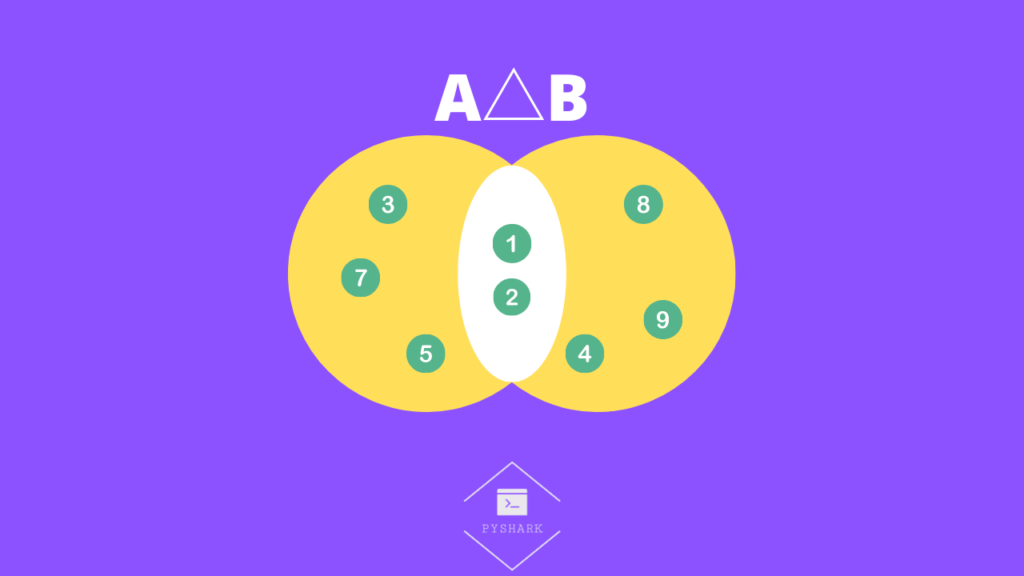In this case: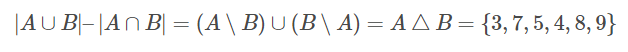Step 2:

The second step is to find the set union of A and B :In this case:

$A cup B = {1, 2, 3, 5, 7, 4, 8, 9}$

Step 3:

And the final step is to take the ratio of sizes of symmetric difference and union: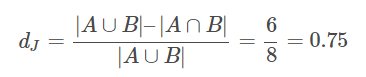Similarity and distance of asymmetric binary attributes

In this section we will look into a more specific application of Jaccard similarity and Jaccard distance. More specifically, their application to asymmetric binary attributes.

From the naming of it, we can already guess what a binary attribute is. It’s an attribute that has only two states, and those two states are:

• 0, meaning an attribute is not present
• 1, meaning an attribute is present

The asymmetry comes from the point that if both attributes are present (both equal to 1), it is considered more important, than if both attributes weren’t present (both equal to 0).

Suppose we have two vectors, A and B , each with (n) binary attributes.

In this case, the Jaccard similarity (index) can be calculated as: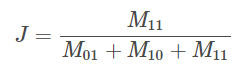and Jaccard distance can be calculated as: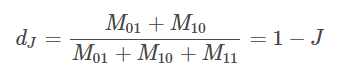where:

• $M_{11}$

• $M_{01}$

• $M_{10}$

• $M_{00}$

and: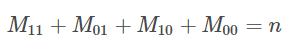Example

To explain this in more simple terms, consider the example that can be used for market basket analysis.

You operate a store that has 6 products (attributes) and 2 customers (objects), and also keep track of which customer bought which item. You know that:

• Customer A bought: apple, milk coffee
• Customer B bought: eggs, milk, coffee

As you can already imagine, we can construct the following matrix: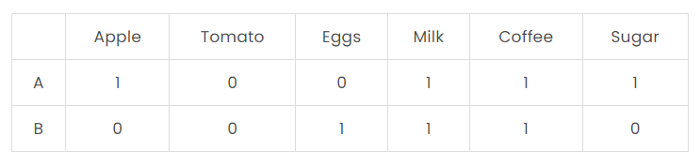Where the binary attribute for each customer is indicating if customer purchased (1) or didn’t purchase (0) a particular product.

The question is to find the Jaccard similarity and Jaccard distance for these two customers.

Step 1:

We will first need to find the total number for attributes for each

$M$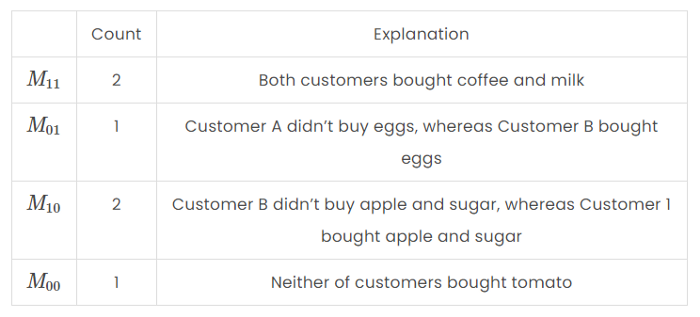We can validate the groups by summing up the counts. it should be equal to 6 which is the

$n$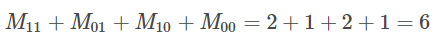Step 2:

Since we have all the required inputs, we can now calculate the Jaccard similarity: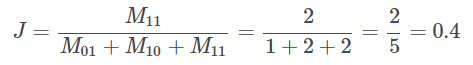And Jaccard distance: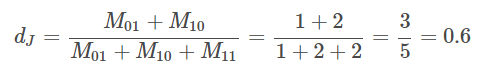Calculate Jaccard similarity in Python

In this section we will use the same sets as we defined in the one of the first sections:

• A = {1, 2, 3, 5, 7}
• B = {1, 2, 4, 8, 9}

We begin by defining them in Python:

A = {1, 2, 3, 5, 7}
B = {1, 2, 4, 8, 9}

As the next step we will construct a function that takes set A and set B as parameters and then calculates the Jaccard similarity using set operations and returns it:

def jaccard_similarity(A, B):
#Find intersection of two sets
nominator = A.intersection(B)

#Find union of two sets
denominator = A.union(B)

#Take the ratio of sizes
similarity = len(nominator)/len(denominator)

return similarity

Then test our function:

similarity = jaccard_similarity(A, B)

print(similarity)

And you should get:

0.25

which is exactly the same as the statistic we calculated manually.

Calculate Jaccard distance in Python

In this section we continue working with the same sets ( A and B ) as in the previous section:

• A  = {1, 2, 3, 5, 7}
• B  = {1, 2, 4, 8, 9}

We begin by defining them in Python:

A = {1, 2, 3, 5, 7}
B = {1, 2, 4, 8, 9}

As the next step we will construct a function that takes set A and set B as parameters and then calculates the Jaccard similarity using set operations and returns it:

def jaccard_distance(A, B):
#Find symmetric difference of two sets
nominator = A.symmetric_difference(B)

#Find union of two sets
denominator = A.union(B)

#Take the ratio of sizes
distance = len(nominator)/len(denominator)

return distance

distance = jaccard_distance(A, B)

Then test our function:

distance = jaccard_distance(A, B)

print(distance)

And you should get:

0.75

which is exactly the same as the statistic we calculated manually.

Calculate similarity and distance of asymmetric binary attributes in Python

We begin by importing the required dependencies:

import numpy as np
from scipy.spatial.distance import jaccard
from sklearn.metrics import jaccard_score

Using the table we used in the theory section:we can create the required binary vectors:

A = np.array([1,0,0,1,1,1])
B = np.array([0,0,1,1,1,0])

and then use the libraries’ function to calculate the Jaccard similarity and Jaccard distance:

similarity = jaccard_score(A, B)
distance = jaccard(A, B)

print(f'Jaccard similarity is equal to: {similarity}')
print(f'Jaccard distance is equal to: {distance}')

And you should get:

Jaccard similarity is equal to: 0.4
Jaccard distance is equal to: 0.6

which is exactly the same as the statistic we calculated manually.

Conclusion

In this article we explored Jaccard similarity (index) and Jaccard distance as well as how to calculate them in Python.

Feel free to leave comments below if you have any questions or have suggestions for some edits and check out more of my Statistics articles.